## RS Aggarwal Class 7 Solutions Chapter 7 Linear Equations in One Variable Ex 7C

These Solutions are part of RS Aggarwal Solutions Class 7. Here we have given RS Aggarwal Solutions Class 7 Chapter 7 Linear Equations in One Variable Ex 7C.

Other Exercises

Objective Questions :
Mark (✓) against the correct answer in each of the following :
Question 1.
Solution:
(d)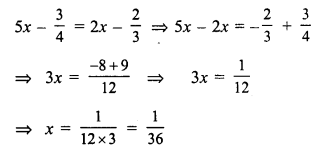Question 2.
Solution:
(d)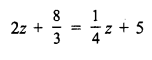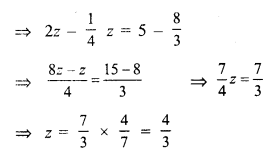Question 3.
Solution:
(a)
2n + 5 = 3 (3n – 10)
⇒ 2n + 5 = 9n – 30
⇒ 9n – 2n = 5 + 30
⇒ 7n = 35
⇒ n = 5

Question 4.
Solution:
(c)Question 5.
Solution:
(c)
8 (2x – 5) – 6 (3x – 7) = 1
⇒ 16x – 40 – 18x + 42 = 1
⇒ -2x + 2 = 1
⇒ -2x = 1 – 2 = -1
x = $$\frac { 1 }{ 2 }$$

Question 6.
Solution:
(d)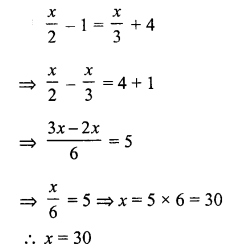Question 7.
Solution:
(a)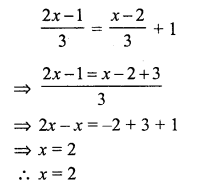Question 8.
Solution:
(b)
Let first whole number=x
Then second number = x + 1
and sum = 53
x + x + 1 = 53
⇒ 2x = 53 – 1
⇒ 2x = 52
⇒ x = 26
Smaller number = 26

Question 9.
Solution:
Let first even number = 2x
Then second number = 2x + 2
and sum = 86
2x + 2x + 2 – 86
⇒ 4x = 86 – 2 = 84
⇒ x = 21
Larger even number = 2x + 2 = 2 x 21 + 2 = 42 + 2 = 44

Question 10.
Solution:
(b)
Let first odd number = 2x + 1
Second number = 2x + 3
2x + 1 + 2x + 3 = 36
⇒ 4x + 4 = 36
⇒ 4x = 36 – 4 = 32
⇒ x = 8
Smaller number = 2x + 1 = 2 x 8 + 1 = 16 + 1 = 17

Question 11.
Solution:
(d)
Let number = x
2x + 9 = 31
⇒ 2x = 31 – 9 = 22
⇒ x = 11

Question 12.
Solution:
(a)
Let number = x then
3x + 6 = 24
⇒ 3x = 24 – 6 = 18
⇒ x = 6
Number = 6

Question 13.
Solution:
(a)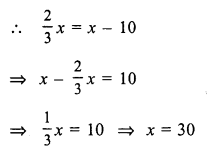Question 14.
Solution:
(b)
Let first angle = x
Then second = 90° – x
x – (90° – x) = 10
⇒ x – 90° + x = 10°
⇒ 2x = 10° + 90° = 100°
x = 50°
Second angle = 90° – 50° = 40°
Larger angle = 50°

Question 15.
Solution:
(b)
Let first angle = x
Then second = 180° – x
x – (180° – x) = 20°
⇒ x – 180° + x = 20°
⇒ 2x = 20° + 180° = 200°
x = 100°
Second angle = 180° – 100° = 80°
Smaller angle = 80°

Question 16.
Solution:
(c)
Let age of A = 5x
Then age of B = 3x
After 6 years,
A’s age = 5x + 6
and B’s age = 3x + 6
$$\frac { 5x + 6 }{ 3x + 6 }$$ = $$\frac { 7 }{ 5 }$$
⇒ 25x + 30 = 21x + 42
⇒ 25x – 21x = 42 – 30
⇒ 4x = 12
⇒ x = 3
A’s age = 5x = 5 x 3 = 15 years

Question 17.
Solution:
(b)
Let the number = x
According to the condition,
5x = 80 + x
⇒ 5x – x = 80
⇒ 4x = 80
⇒ x = 20
Number = 20

Question 18.
Solution:
(c)
Let width of rectangle = x m
Then length = 3x m
Perimeter = 96 m
2 (x + 3x) = 96
⇒ x + 3x = $$\frac { 96 }{ 2 }$$ = 48
⇒ 4x = 48
⇒ x = 12
Length = 3x = 12 x 3 = 36 m

Hope given RS Aggarwal Solutions Class 7 Chapter 7 Linear Equations in One Variable Ex 7C are helpful to complete your math homework.

If you have any doubts, please comment below. Learn Insta try to provide online math tutoring for you.